Author: Oscar Cronquist Article last updated on January 08, 2023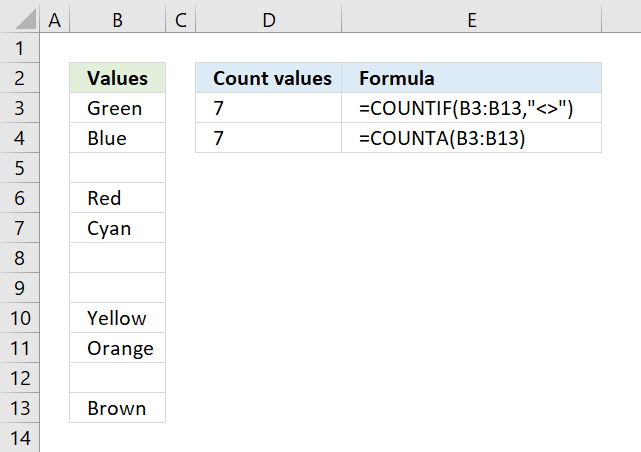The COUNTIF function is very capable of counting non-empty values, I will show you how in this article. Excel can also highlight empty cells using Conditional formatting.

I will discuss and demonstrate the limitations of using the COUNTIF function and other equivalent functions that you also can use.

## 1. Count not blank cells - COUNTIF functionColumn B above has a few blank cells, they are in fact completely empty.

Formula in cell D3:

=COUNTIF(B3:B13,"<>")

The first argument in the COUNTIF function is the cell range where you want to count matching cells to a specific value, the second argument is the value you want to count.

COUNTIF(range, criteria)

In this case, it is "<>" meaning not equal to and then nothing, so the COUNTIF function counts the number of cells that are not equal to nothing. In other words, cells that are not empty.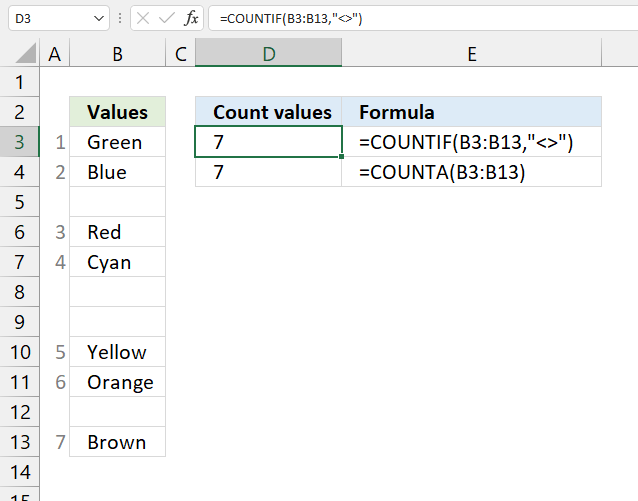## 2. Count not blank cells - COUNTA functionThe COUNTA function is even easier to use, you don't need to enter more than the cell range in one argument. The COUNTA function is designed to count non-empty cells.

COUNTA(value1, [value2], ...)

Formula in cell D4:

=COUNTA(B3:B13)

## 3. COUNTIF and COUNTA function return unexpected results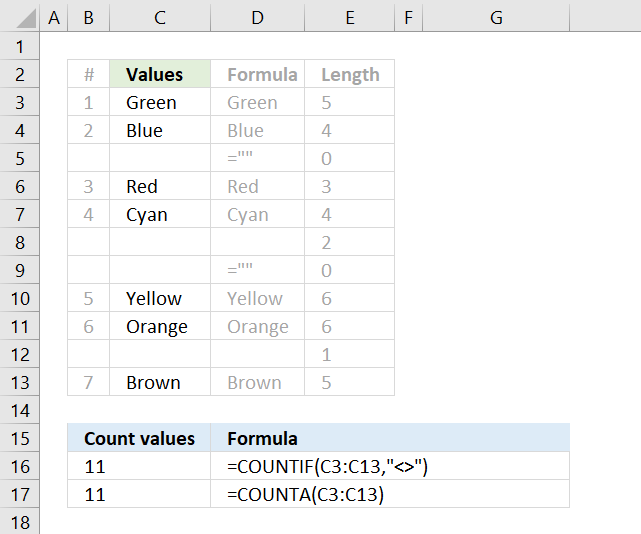There are, however, situations where the COUNTIF and COUNTA function return unexpected results if you are not aware of how they work.

There are blank cells in column C, shown in the picture above, that look empty but they are not. Column D shows what they actually contain and column E shows the character length of the content.

Cell C5 and C9 contain a formula that returns a blank, both the COUNTIF and the COUNTA function count those cells as non-empty.

Cell C8 has two space characters and cell C12 has one space character, column E reveals their existence by counting character length. The COUNTIF and the COUNTA function count those cells as non-empty as well.

## 4. Count not blank cells - SUMPRODUCT function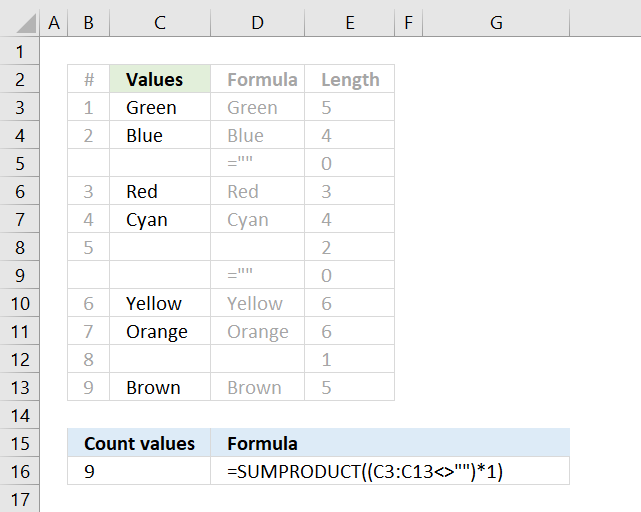The following formula counts all non-empty values in cell range C3:C13 except formulas that return nothing. It checks if the values in cell range C3:C13 are not equal to nothing.

Formula in cell B16:

=SUMPRODUCT((C3:C13<>"")*1)

### 4.1 Explaining formula in cell B16

#### Step 1 - Check if cells are not empty

In this case, the logical expression counts cells that contain space characters but not formulas that return nothing.

The less than and the greater than characters are logical operators, the result are always boolean values.

C3:C13<>""

returns

{TRUE; TRUE; FALSE; TRUE; TRUE; TRUE; FALSE; TRUE; TRUE; TRUE; TRUE}

#### Step 2 - Convert boolean values

The SUMPRODUCT function can't sum boolean values, we need to multiply with one to create an array containing 0's (zero) and 1's.

They are their numerical equivalents:
True = 1
FALSE = 0 (zero)

(C3:C13<>"")*1

becomes

{TRUE; TRUE; FALSE; TRUE; TRUE; TRUE; FALSE; TRUE; TRUE; TRUE; TRUE}*1

and returns

{1; 1; 0; 1; 1; 1; 0; 1; 1; 1; 1}

#### Step 3 - Sum numbers

Why use the SUMPRODUCT function and not the SUM function? The SUMPRODUCT function can perform calculations in the arguments without the need to enter the formula as an array formula.

Array formulas are great but if possible avoid as much as you can. Excel 365 users don't have this problem, dynamic array formulas are entered as regular formulas.

SUMPRODUCT((C3:C13<>"")*1)

becomes

SUMPRODUCT({1; 1; 0; 1; 1; 1; 0; 1; 1; 1; 1})

and returns 9 in cell B16.

## 5. Regard formulas that return nothing to be blank and space characters to also be blank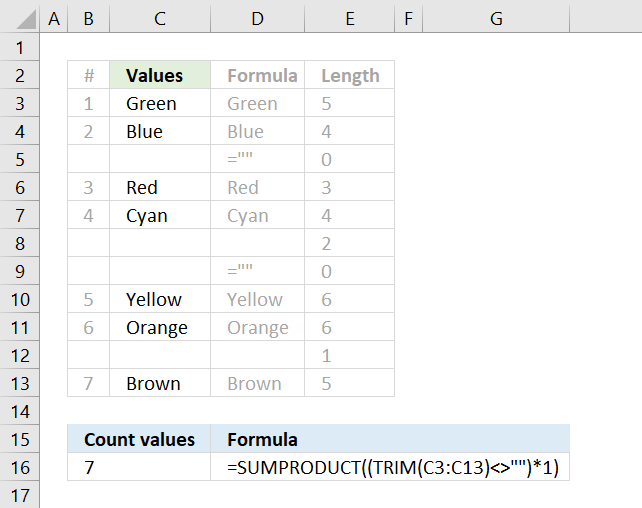The formula above in cell C16 counts only non-empty values, it considers formulas that return nothing to be blank and space characters to also be blank. This is made possible by the TRIM function that removes leading and ending space characters.

=SUMPRODUCT((TRIM(C3:C13)<>"")*1)

### 5.1 Explaining formula in cell B16

#### Step 1 - Remove space characters

TRIM(C3:C13)

returns

{"Green"; "Blue"; ""; "Red"; "Cyan"; ""; ""; "Yellow"; "Orange"; ""; "Brown"}

#### Step 2 - Identify not blank cells

TRIM(C3:C13)<>""

becomes

{"Green"; "Blue"; ""; "Red"; "Cyan"; ""; ""; "Yellow"; "Orange"; ""; "Brown"}<>""

and returns

{TRUE; TRUE; FALSE; TRUE; TRUE; FALSE; FALSE; TRUE; TRUE; FALSE; TRUE}

#### Step 3 - Multiply with 1

TRIM(C3:C13)<>"")*1

becomes

{TRUE; TRUE; FALSE; TRUE; TRUE; FALSE; FALSE; TRUE; TRUE; FALSE; TRUE}*1

and returns

{1; 1; 0; 1; 1; 0; 0; 1; 1; 0; 1}

#### Step 4 - Sum numbers in array

SUMPRODUCT((TRIM(C3:C13)<>"")*1)

becomes

SUMPRODUCT({1; 1; 0; 1; 1; 0; 0; 1; 1; 0; 1})

and returns 7.